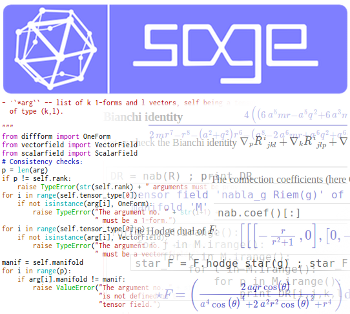## News:

Pinned:

### SageManifolds project

The SageManifolds project aims at extending the modern Python-based computer algebra system SageMath towards differential geometry and tensor calculus. All SageManifolds code is included in SageMath, i.e. it does not require any separate installation.

SageManifolds deals with differentiable manifolds of arbitrary dimension. Various coordinate charts and vector frames can be introduced on the manifold, which does not need to be parallelizable. A given tensor field is then described by its sets of components in each vector frame, with automatic change-of-frame transformations for overlapping vector frames.

Generic pseudo-Riemannian manifolds can be considered, among which Riemannian manifolds and Lorentzian manifolds, with applications to General Relativity. In particular, the computation of the Riemann curvature tensor and associated tensors (Ricci, Weyl, Schouten and Cotton tensors) is implemented. SageManifolds can also deal with generic affine connections, not necessarily Levi-Civita ones.

At present (SageMath 10.1), the functionalities include:

• topological manifolds: charts, open subsets, maps between manifolds, scalar fields
• differentiable manifolds: tangent spaces, vector frames, tensor fields, curves, pullback and pushforward operators
• vector bundles
• standard tensor calculus (tensor product, contraction, symmetrization, etc.), even on non-parallelizable manifolds
• all monoterm tensor symmetries
• p-forms (exterior product, interior product with p-vectors, exterior derivative, Hodge duality)
• p-vectors (exterior product, interior product with p-forms, Schouten-Nijenhuis bracket)
• mixed differential forms
• Lie derivatives of tensor fields
• series expansion of tensor fields
• affine connections (curvature, torsion)
• pseudo-Riemannian metrics
• computation of geodesics
• some plotting capabilities (charts, points, curves, vector fields)
• submanifolds and their extrinsic geometry
• nilpotent Lie groups
• de Rham cohomology and characteristic classes
• symplectic structures (symplectic form, Poisson bivector, musical isomorphisms, Hamiltonian vector fields, Poisson brackets, Liouville volume form, symplectic Hodge dual)

For an overview, see the tutorial or the example notebooks. To stay tuned, subscribe to the mailing list.

### Open and free software

As part of SageMath, all SageManifolds code is free and open source; it is released under the GNU General Public License. To download it, see here.

SageManifolds is developed by these authors.

If you want to take part in the project, visit the contribute page, or if you simply want to stay tuned, subscribe to the mailing list.